• 品质因数 物理意义 电感线圈、电容器品质因数：用于评价实际电感线圈、电容器的品质。实际电感、电容除储存能量外，都存在一定能量消耗。 元件品质因数定义：Q=def2π储能的最大值一周期内的耗能 Q \overset{\...
品质因数

物理意义

电感线圈、电容器品质因数：用于评价实际电感线圈、电容器的品质。实际电感、电容除储存能量外，都存在一定能量消耗。
元件品质因数定义：$Q \overset{\underset{\mathrm{def}}{}}{=} 2\pi\frac{储能的最大值}{一周期内的耗能}$
品质因数用来衡量元件质量的好坏，将品质因数扩展到谐振电路，表示谐振电路储能与耗能的比值。

电感线圈的品质因数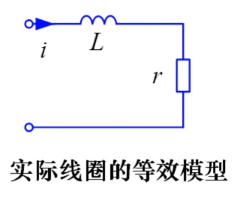线圈电流：$i = \sqrt{2}Icos\omega t$
电感储能：$w_{L}(t)=\frac{1}{2}Li^{2}=LI^{2}cos^{2}\omega t$
最大储能为：$LI^{2}$
一周期内线圈内阻r的耗能：$I^{2}rT$
电感品质因数：$Q_{L}=2\pi\frac{LI^{2}}{I^{2}rT}=\frac{2\pi fL}{r} =\frac{\omega L}{r}$

电容的品质因数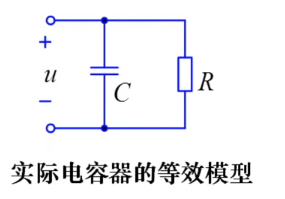电容电压：$u=\sqrt{2}Ucos\omega t$
电容储能：$w_{C}(t)=\frac{1}{2}Cu^{2}=CU^{2}cos^{2}\omega t$
最大储能：$CU^{2}$
一周期电阻R耗能：$\frac{U^{2}}{R}T$
电容品质因数：$Q_{C}=2\pi\frac{CU^{2}}{U^{2}GT}=\frac{2\pi fC}{G} =\frac{\omega C}{G}=\omega CR$

rLC串联谐振电路中的品质因数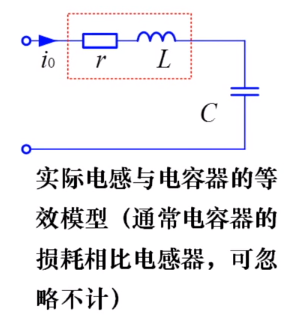电路谐振时，回路电流：$i=\sqrt{2}cos\omega _{0}t$
电容电压相量：$\dot{U_{C0}}=-j\frac{1}{\omega _{0}C}\dot{I_{0}}=\frac{I_{0}}{\omega _{0}C}\angle 90^{o}$
电容电压瞬间式：$u_{c0}=\sqrt{2}\frac{I_{0}}{\omega _{0}C}cos(\omega _{0}t-90^{o})=\sqrt{2}\frac{I_{0}}{\omega _{0}C}sin(\omega _{0}t)$
电感储能：$w_{L0}(t)=\frac{1}{2}Li_{L0}^{2}=LI_{0}^{2}cos^{2}\omega_{0}t$
电容储能：$w_{C0}(t)=\frac{1}{2}Cu_{C0}^{2}=C(\frac{I_{0}}{w_{0}C})^{2}sin^{2}\omega_{0} t$
条件：谐振电路中$\omega_{0}L=\frac{1}{\omega_{0}C}$
可得电容储能等于电感储能：$w_{C0}(t)=C(\frac{I_{0}}{w_{0}C})^{2}sin^{2}\omega_{0} t=LI^{2}sin^{2}\omega_{0} t$
谐振电路的总储能为电感储能与电容储能之和：$w_{0}(t)=w_{L0}(t) +w_{C0}(t)=LI^{2}_{0}=CU^{2}_{0},(常数)$
当电感储能最大时电容储能为零，反之亦然。
谐振电路谐振时的品质因数：$Q=2\pi\frac{LI^{2}_{0}}{I^{2}_{0}rT_{0}}=\frac{w_{0}L}{r}=\frac{1}{w_{0}Cr}$
谐振电路品质因数反映电路选频特性，品质因数越高，选频特性越好。

小结

品质因数反映器件和电路的特性。
元件串联时，谐振电路品质因数和电阻成反比，电阻越大，品质因数越低。
品质因数是衡量谐振电路优势的一个重要参数。


展开全文•研究论文
• 品质因数是谐振电路中一个非常重要的参数, 然而目前国内一般教材对品质因数的定义和计算方 法, 仅限于在简单 RLC 串、并联谐振电路的过压、过流方面
•研究论文
•研究论文
• ## 电路品质因数Q

万次阅读 2019-05-19 11:33:48
品质因数Q=ULUsQ=\frac{ U_L}{U_s}Q=Us​UL​​,即电感上的电压比上输入电压,是分析和比较谐振电路的一个重要辅助参数。 不同参数的RLC电路在频率响应上的差异，是通过Q值反映出来的。在谐振电路中，它们有以下不同...

品质因数$Q=\frac{ U_L}{U_s}$,即电感上的电压比上输入电压,是分析和比较谐振电路的一个重要辅助参数。
不同参数的RLC电路在频率响应上的差异，是通过Q值反映出来的。在谐振电路中，它们有以下不同点
1.它们都在谐振点出现峰值，在其领域都有较大的幅值输出，表明RLC串联电路具有在全频域内选择各自频振信号的性能；
2.当信号频率偏移谐振点，输出信号从峰值下降，表明信号对非谐振频率有抑制作用（简称抑非能力）。抑非能力与Q值成正比，能力越强，通频带越窄，电路选择性越好；能力越弱，通频带越宽，电路选择性变差。

二阶传递函数的品质因数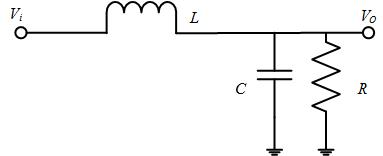传递函数$G={\frac{V_o}{V_i}}={\frac{1}{S^2LC+SL/R+1}}$
品质因数$Q=R\sqrt{\frac{C}{L}}$
LC滤波器伯德图如下图所示：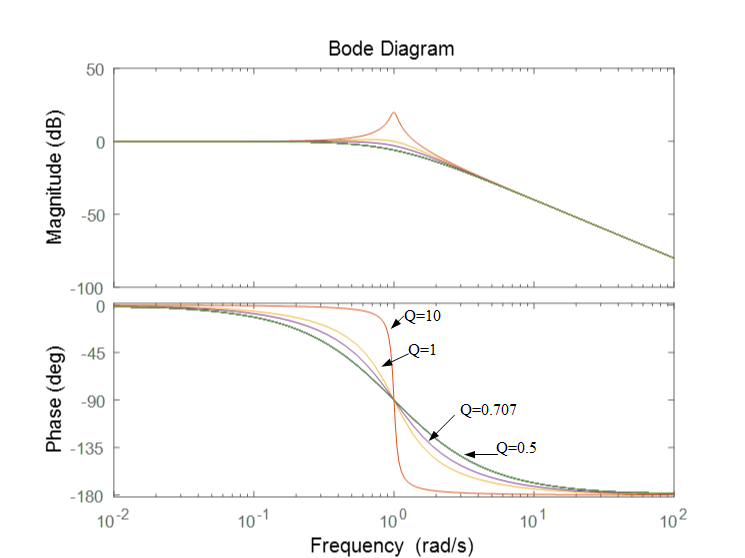品质因数Q确定了响应曲线在交接频率处的峰值大小，如图Q=20,那么谐振下输出电压是输入电压的20倍。一般认为Q值越大，则滤波器欠阻尼状态，当Q=0.707，得到临界阻尼，谐振频率的增益比其直流值低3dB。-3dB是0.707的对数，意味着下降约30%。
电阻对交接频率影响较小，但其对Q的影响是显著地。L和C的串联寄生电阻越大，Q值越小。
工程师一般用阻尼系数 $ξ={\frac{1}{2Q}}$,高Q值对应小ξ。


展开全文• 提出了一种以非对称双开口环谐振器为基本单元的新型高品质因数Q 太赫兹超材料结构。当入射电场为垂直极化时，所提出的结构在不同的太赫兹频段分别表现出束缚模谐振、混合模式谐振和偶极子谐振3 种谐振响应，通过改变...
• ## 品质因数Q

千次阅读 2012-10-14 10:37:53
中文名称：品质因数 英文名称：quality factor，Q factor 定义：无功功率的绝对值与有功功率之比，即损耗角正切的倒数。 应用学科：电力（一级学科）；通论（二级学科） 以上内容由全国科学技术名词审定委员会...

科技名词定义
中文名称：品质因数 英文名称：quality factor，Q factor 定义：无功功率的绝对值与有功功率之比，即损耗角正切的倒数。 应用学科：电力（一级学科）；通论（二级学科）
以上内容由全国科学技术名词审定委员会审定公布

目录

简介
计算
提高品质因数的措施

编辑本段简介
品质因数(Q因数)

quality factor

电学和磁学的量。表征一个储能器件(如电感线圈、电容等)、谐振电路中所储能量同每周期损耗能量之比的一种质量指标；电抗元件的Q值等于它的电抗与其等效串联电阻的比值；元件的Q值愈大,用该元件组成的电路或网络的选择性愈佳。

编辑本段计算
对于无辐射系统,如Z=R+jX,则Q =|X|/R。SI单位:1(一)。

Q=无功功率/有功功率

谐振回路的品质因数为谐振回路的特性阻抗与回路电阻之比。

在串联电路中，电路的品质因数Q有两种测量方法，一是根据公式 Q=UL/U0=Uc/U0测定，Uc与UL分别为谐振时电容器C与电感线圈L上的电压；另一种方法是通过测量谐振曲线的通频带宽度△f=f2-f1，再根据Q=f0/(f2-f1)求出Q值。式中f0为谐振频率，f2与f1是失谐时，亦即输出电压的幅度下降到最大值的1/√2（=0.707)倍时的上、下频率点。Q值越大，曲线越尖锐，通频带越窄，电路的选择性越好。在恒压源供电时，电路的品质因数、选择性与通频带只决定于电路本身的参数，与信号源无关。

1是一串联谐振电路，它由电容C、电感L和由电容的漏电阻与电感的线电阻R所组成。此电路的复数阻抗Z为三个 元件的复数阻抗之和。

Z=R+jωL+(-j/ωC)=R+j(ωL-1/ωC) ⑴

上式电阻R是复数的实部，感抗与容抗之差是复数的虚部，虚部我们称之为电抗用X表示, ω是外加信号的角频率。当X=0时，电路处于谐振状态,此时感抗和容抗相互抵消了，即式⑴中的虚部为零，于是电路中的阻抗最小。因此电流最大，电路此时是一个纯电阻性负载电路，电路中的电压与电流同相。电路在谐振时容抗等于感抗，所以电容和电感上两端的电压有效值必然相等，电容上的电压有效值UC=I*1/ωC=U/ωCR=QU 品质因素Q=1/ωCR，这里I是电路的总电流。

电感上的电压有效值UL=ωLI=ωL*U/R=QU 品质因素Q=ωL/R

因为：UC=UL 所以Q=1/ωCR=ωL/R

电容上的电压与外加信号电压U之比UC/U= （I*1/ωC）/RI=1/ωCR=Q

感上的电压与外加信号电压U之比UL/U= ωLI/RI=ωL/R=Q

从上面分析可见，电路的品质因素越高，电感或电容上的电压比外加电压越高。

电路的选择性：图1电路的总电流I=U/Z=U/[R2+(ωL-1/ωC)2]1/2=U/[R2+(ωLω0/ω0-ω0/ωCω0)2]1/2 ω0是电路谐振时的角频率。当电路谐振时有：ω0L=1/ω0C

所以I=U/{R2+[ω0L(ω/ω0-ω0/ω)]2}1/2= U/{R2+[R2(ω0L/R)2](ω/ω0-ω0/ω)2}1/2= U/R[1+Q2(ω/ω0-ω0/ω)2]1/2

因为电路谐振时电路的总电流I0=U/R，

所以I=I0/[1+Q2(ω/ω0-ω0/ω)2]1/2有：I/I0=1/[1+Q2(ω/ω0-ω0/ω)2]1/2作此式的函数曲线。设(ω/ω0-ω0/ω)2=Y

曲线如图2所示。这里有三条曲线，对应三个不同的Q值，其中有Q1>Q2>Q3。从图中可看出当外加信号频率ω偏离电路的谐振频率ω0时， I/I0均小于1。Q值越高在一定的频偏下电流下降得越快，其谐振曲线越尖锐。也就是说电路的选择性是由电路的品质因素Q所决定的，Q值越高选择性越好。

编辑本段提高品质因数的措施
①根据工作频率选择绕制线圈的导线。低频段工作的电感线圈应采用漆包线等带绝缘的导线绕制。对于工作频率在几十千赫至两兆赫之间的电感线圈，应采用多股绝缘导线绕制，以增加导体有效截面积，减少集肤效应的影响，可使Q值提高30%-40%。对于工作频率高于2MHz的电感线圈，应采用单股粗导线绕制，导线的直径一般在0.3-1.5mm之间。

②选用优质骨架，减少介质损耗。通常对于要求损耗小、工作频率高的电感线圈，应选用高频陶瓷、聚四氟乙烯、聚苯乙烯等高频介质材料做骨架。对于超高频工作的电感线圈，可用无骨架方式绕制。

③选用带有磁心的电感线圈。电感线圈中带有磁心时，可使线圈圈数及其电阻大大减少，有利于Q值的提高。

④合理选择屏蔽罩的尺寸。线圈加屏蔽罩后，会增加线圈的损耗，降低Q值。因此，屏蔽罩的尺寸不宜过大和过小。一般来说，屏蔽罩直径与线圈直径之比以1.6-2.5为宜，这样可使Q值降低小于10%。

参考资料：电路品质因数的计算http://wenku.baidu.com/view/0c0b7a5e804d2b160b4ec020.html

展开全文c 网络
• 以提高傅里叶变换光谱仪的光谱分辨率，在建立弹光调制器的损耗模型和振动方程的基础上，描述了包含损耗因数的弹光调制器幅频特性，并推导了谐振和反谐振频率下弹光调制器的品质因数。结合压电材料的电致伸缩效应及其...
• 针对基于谐波电压或阻抗的孤岛检测方法所存在的盲区较大且阈值难以设定的问题，提出一种基于不同次数谐波下品质因数一致性的孤岛检测判据，并结合q轴谐波电流扰动法作为新型孤岛检测方案。推导了微网发生孤岛后负载...
•研究论文
• 电感电桥主要用来测量线圈的电感及其品质因数。多种线路，大都采用标准电容作为与被测电感相比较的标准元件。 　实际电感线圈可用电阻R与理想电感L的串联等效电路来代替。品质因数Q是指线圈电阻R与感抗ωL的比值，...
• 基于Fano共振的高品质因数近红外等离子体滤波器的数值分析。研究论文
• 高真空密封MEMS陀螺仪的封装可靠性和品质因数退化模型研究研究论文
• 在学习通信电子线路的过程中，起初只是把品质因数（品质因子Q）理解成一个人为规定好的无量纲参数，并未再多关注。 实际上，品质因子是物理、工程上衡量振子阻尼性质的物理量和振子的共振频率相对于带宽的大小的重要...
在学习通信电子线路的过程中，起初只是把品质因数（品质因子Q）理解成一个人为规定好的无量纲参数，并未再多关注。
实际上，品质因子是物理、工程上衡量振子阻尼性质的物理量和振子的共振频率相对于带宽的大小的重要用语。

物理含义

品质因子是表示振子阻尼性质的物理量， 高Q因子表示振子能量损失的速率较慢，振动可持续较长的时间。
例如一个单摆在空气中运动，其Q因子较高，而在油中运动的单摆Q因子较低。
高Q因子的振子一般其阻尼也较小。

电子系统

RLC串联谐振电路中：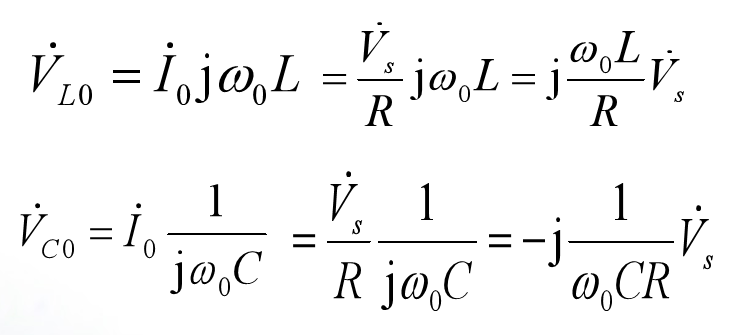为了表征谐振时电感L和电容C两端电压值的大小，
引入电感线圈的品质因数：
$\bm{Q=\frac{wL}{R}}$（线圈的Q值常在几十到一、二百左右）
$\bm{Q也=\frac{1}{wCR}}$ （回路的品质因数：谐振时，回路感抗模（或容抗模）与电阻R的比值）
定义谐振曲线之后（回路中电流幅值与外加电压频率之间的关系曲线），可以用品质因数来更方便的描述广义失谐。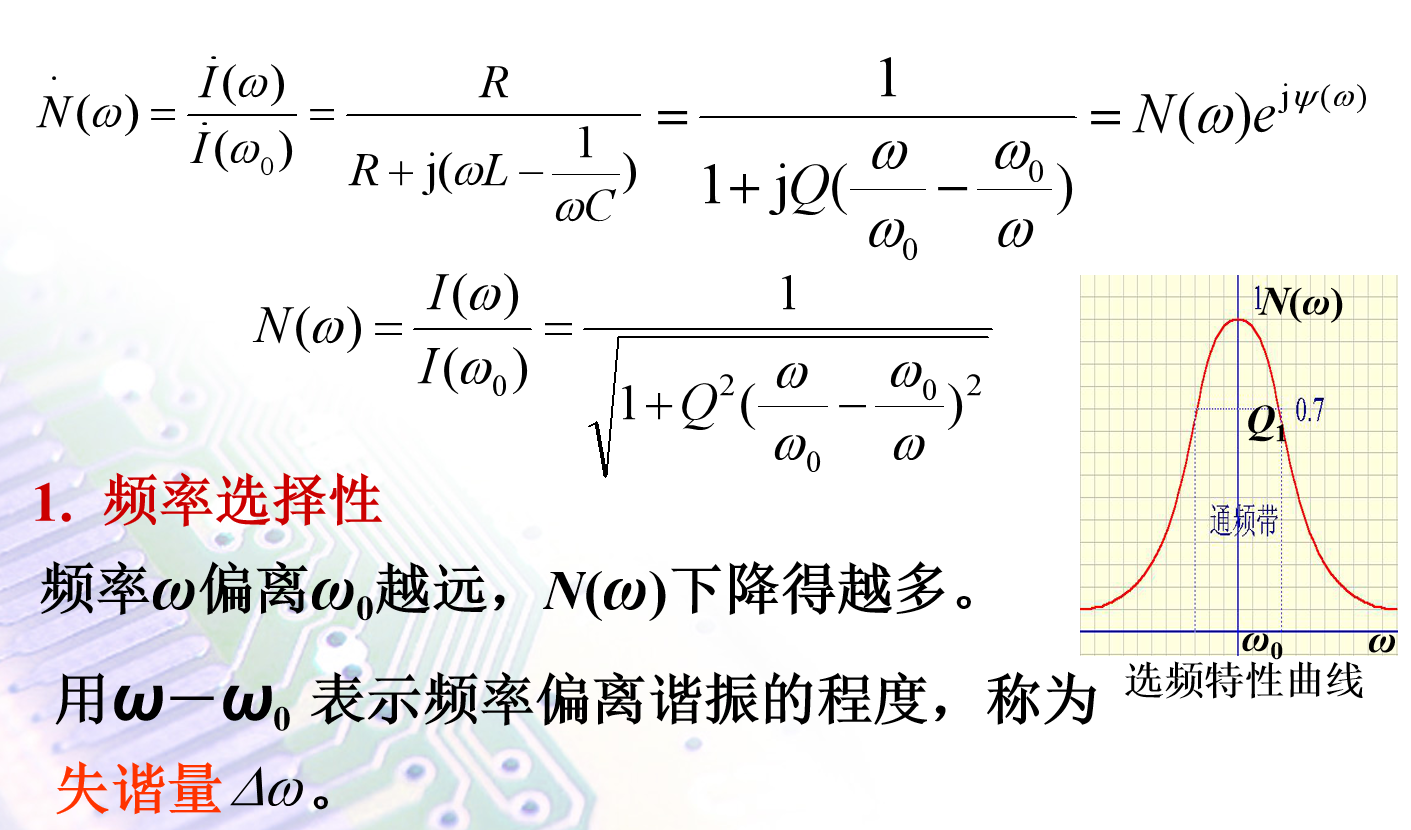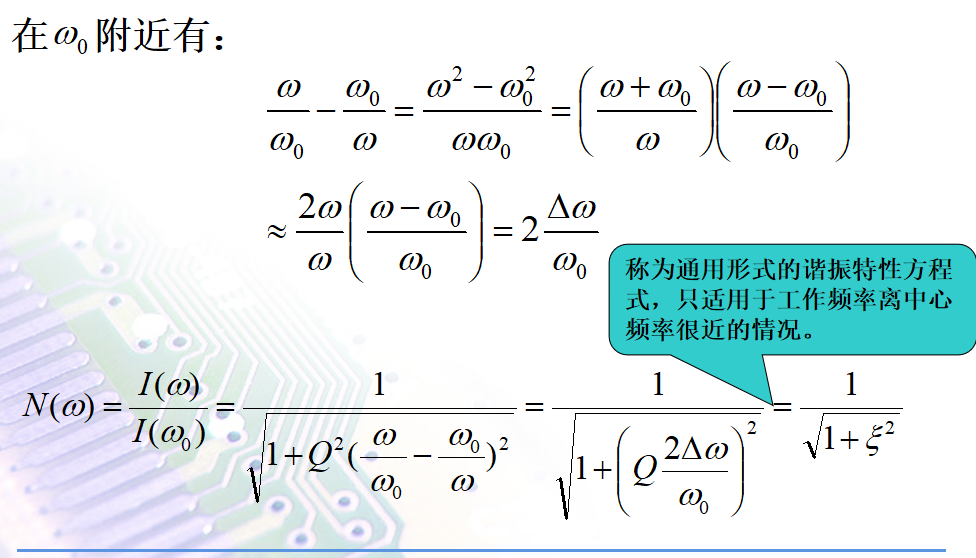当$频率w=w_0时$，失谐量为0，达到谐振状态，谐振时的品质因数$\bm{Q_0=Q=\frac{w_0L}{R}=\frac{1}{w_0CR}}$。
对于同样的频率ω和ω0，回路的Q值愈大， N(ω)下降的越多。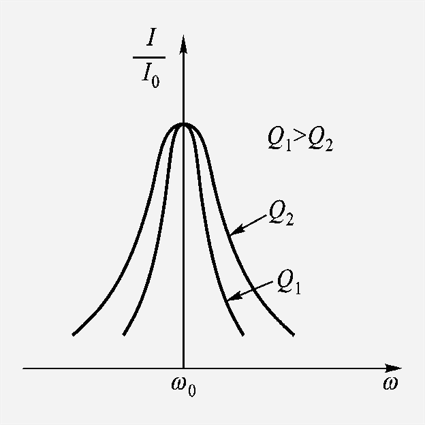RLC并联谐振电路中：
并联RLC电路的Q因子恰为对应串联电路Q因子的倒数。
$\bm{Q=\frac{R}{wL}={wCR}}$
对串联和并联谐振回路而言，谐振曲线也是相似的。

描述电压增益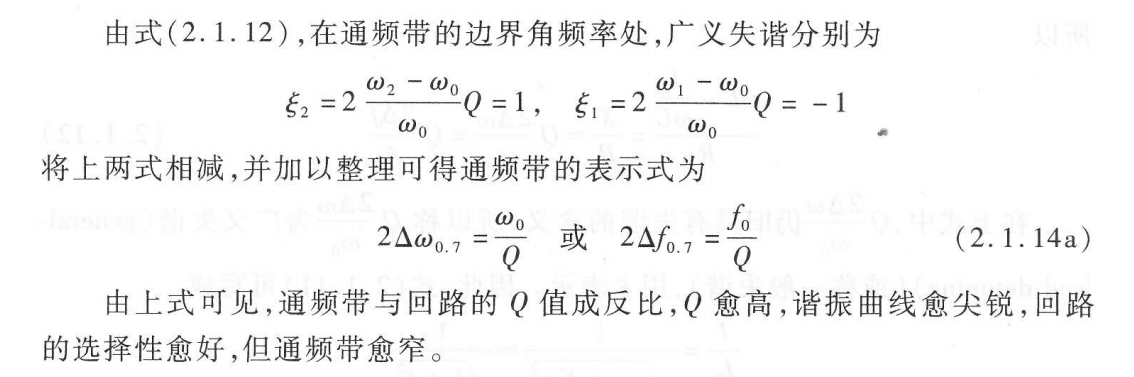将单调谐回路谐振放大器最终等效成以下这样一个RLC并联电路后，我们最关心的就是它的电压增益。
如之前分析的一样，用品质因数$Q$来替换分子中的阻抗值，即替换成广义失谐。
当达到谐振状态时，失谐量$2△f_{0.7}$为0，通频带的相对值为0，分母中的虚数部分为0。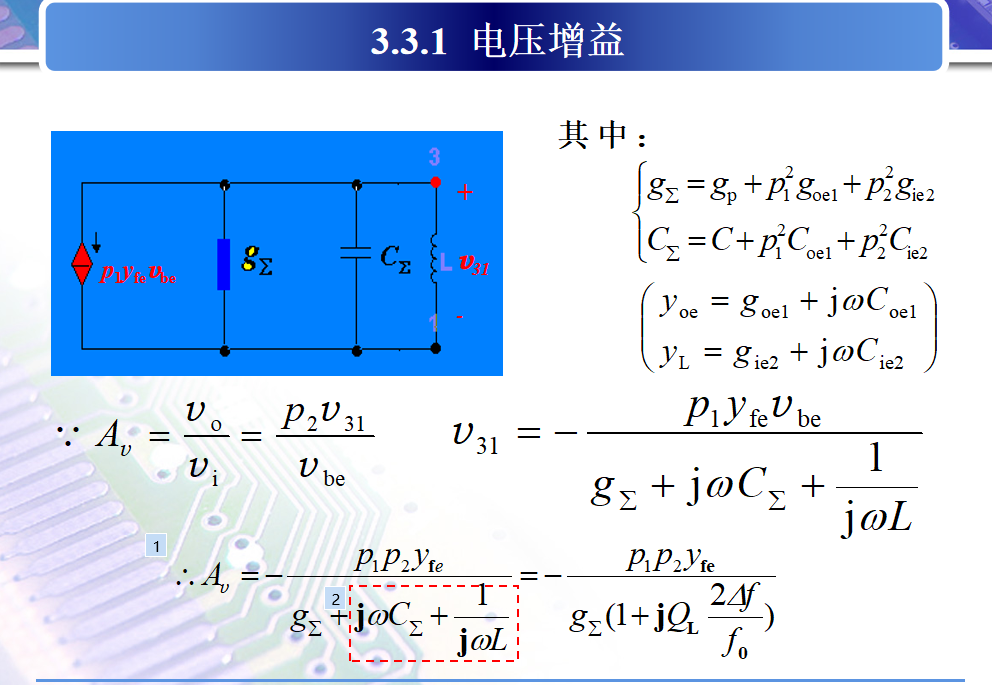在描述滤波特性的曲线当中，也有用到品质因数这样的概念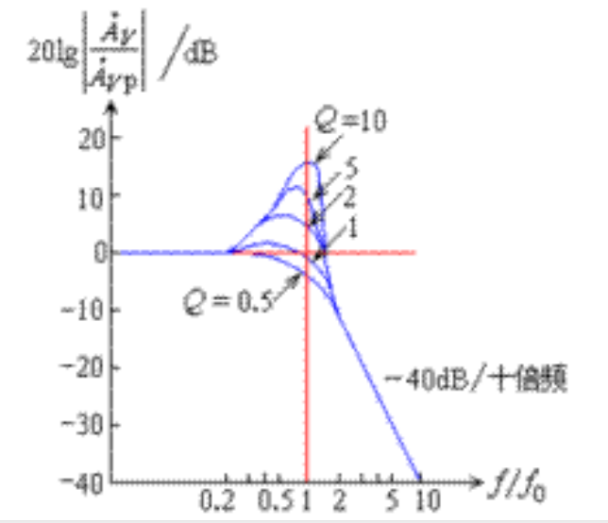不同的Q值。按“最佳逼近特性”标准可分为巴特沃斯滤波器、贝塞尔滤波器、切比雪夫滤波器，它们的Q值分别为0.707、0.56、0.96。


展开全文• 通过仿真与实验探究了提高频率和改善线圈参数两种不同提高系统品质因数的方法对能量传输效率、功率与传输距离之间的影响规律。结果表明，提高系统共振频率可明显提高系统能量有效传输距离，但导致最高输出功率明显...
• L C 电路在调谐放大器和L C 振荡电路等很多电子电路...为了描述L C 回路的性能,人们引入了一个重要概念即品质因数。但一些教材和资料对各种品质因数没有严格分 , 容易使学生产生误解。现对这个问题 , 进行探讨和分析。
• 在金属间隙介电波导上具有超高品质因数的改性表面等离激元极化子研究论文
• 线圈的品质因数称作Q值。它表示线圈在一定频率的交流电压下工作时，其感抗ＸL和等效损耗电阻之比，即为Q值。表示公式为式中：2---常数　f--频率　L--线圈的电感量　R--线圈的总损耗电阻,在低频下可视为线圈的直流...
• 总结： ...但是两种电路的通频带又都反比于品质因数：BW=f0/Q （f0指谐振频率、Q指品质因数）。 品质因数由谐振电路中的电阻R和电感L或电容C控制，可根据实际应用，通过更改R值来更改谐振电路Q值。
• 谐振电路 品质因数Q 不同定义与推导 能量定义 功率定义 能量定义的功率定义相互转换 系数2π的由来
• 品质因数Q的定义首先来讲讲，电感品质因数Q的定义。Q值是衡量电感器件的主要参数，是指电感器在某一频率的交流电压下工作时，所呈现的感抗与其等效损耗电阻之比。电感器的Q值越高，其损耗越小，效率越高。品质因数Q...
• 工程师必懂磁性元件专业术语：初始磁导率、有效磁导率、品质因数、矫顽力、电感因数......1、初始磁导率初始磁导率是磁性材料的磁导率(B/H)在静态磁化曲线始端的极限值，式中(μ)为真空磁导率(4π×10-7H/m)，H为...
• 工程师必懂磁性元件专业术语：初始磁导率、有效磁导率、品质因数、矫顽力、电感因数… 1、初始磁导率 初始磁导率是磁性材料的磁导率（B/H）在静态磁化曲线始端的极限值， 式中（μ）为真空磁导率（4π×10-7H/m）...
• 我们先来说说电容，都说大电容低频特性好,小电容高频特性好，那么根据容抗的大小与电容C及频率F成反比来说的话，是不是大电容不仅低频特性好，高频特性更好呢，因为频率越高，容量越大，容抗就越低，高频就是否越...
• 品质因数介绍： 在研究各种谐振电路时，常常涉及到电路的品质因素Q值的问题，那末什么是Q值呢？下面我们作详细的论述。 1是一串联谐振电路，它由电容C、电感L和由电容的漏电阻与电感的线电阻R所组成。此电路的...
• 品质因数（Q值） 一、Q值的定义 品质因子或Q因子，表示振子阻尼性质的物理量，也可表示振子的共振频率相对于带宽的大小， 高Q因子表示振子能量损失的速率较慢，振动可持续较长的时间。 注：阻尼（damping）是指任何...
• 在研究各种谐振电路时，常常涉及到电路的品质因素Q值的问题，那末什么是Q值呢？本文我们作详细的论述。
• 在研究各种谐振电路时，常常涉及到电路的品质因素Q值的问题，那末什么是Q值呢？下面我们作详细的论述。...